# E-PolyLearning

 1. General crushing equation is given by following equation. Bond's crushing law is obtained by solving this equation for n = __________ and feed of infinite size.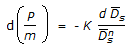a. 1 b. 1.5 c. 2 d. 2.5

 2. According to Bond crushing law, the work required to form particle of size 'D' from very large feed is (where (S/V)p and (S/V)f are surface to volume ratio of the product and feed respectively).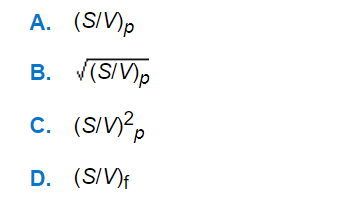a. A b. B c. C d. D
 3. Critical Speed (Nc) of a ball mill is given by(where R₁ and R₂ are radii of ball mill and the ball respectively).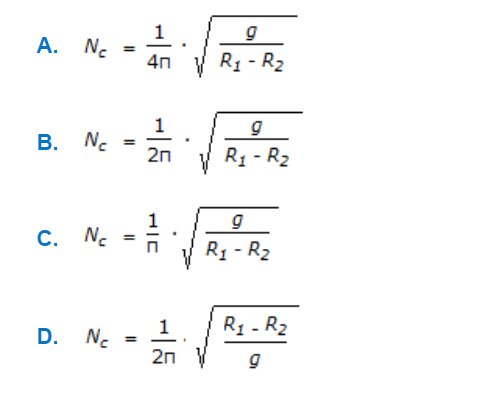a. A b. B c. C d. D
 4. Which of the following equations is Rittinger's crushing law ? (where P = power required by the machine, m = feed rate, k = a constant, D̅sa & D̅sb = volume surface mean diameter of feed & product respectively.)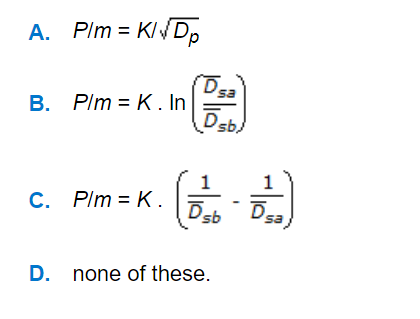a. A b. B c. C d. D
 5. About 2-3 hp, power per gallon of a thin liquid provides vigrous agitation in an agitator. 'Power number' in agitation is given by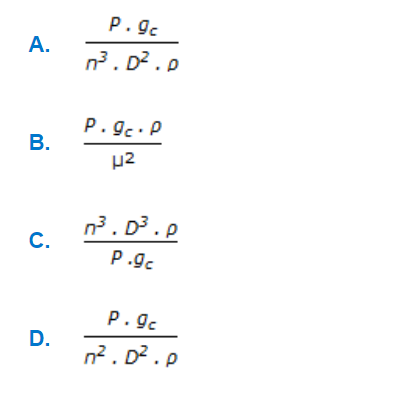a. A b. B c. C d. D